# Introduction

This vignette shows how to use the bayesRecon package for the probabilistic reconciliation of hierarchical forecasts. We provide three examples:

1. Carpart: we build a temporal hierarchy on a count time series and we reconcile it via the Bottom-Up Importance Sampling (BUIS) algorithm.

2. M3: we build a temporal hierarchy on a smooth time series and we reconcile it using Gaussian reconciliation.

3. Infants mortality: we reconcile cross-sectional forecasts.

The implemented reconciliation algorithms are discussed in:

• Zambon, L., Azzimonti, D., & Corani, G. (2022). Efficient probabilistic reconciliation of forecasts for real-valued and count time series.

• Zambon, L., Agosto, A., Giudici, P., & Corani, G. (2023). Properties of the reconciled distributions for Gaussian and count forecasts.

• Corani, G., Azzimonti, D., & Rubattu, N. (2023). Probabilistic reconciliation of count time series.

• Corani, G., Azzimonti, D., Augusto, J. P., & Zaffalon, M. (2021). Probabilistic reconciliation of hierarchical forecast via Bayes’ rule.

# Setup

You can install the stable version of bayesRecon on R CRAN.

install.packages('bayesRecon', dependencies = TRUE)

library(bayesRecon)

# Example 1: Carpart

In this example, we consider a monthly time series of car part sales, from Jan. 1998 to Mar. 2002.
This is actually the time series #2655 of the carparts dataset (R. Hyndman et al. 2008). It is available from bayesRecon::carpart.

The time series consists of count data, and the distribution of its values is skewed. Hence, we produce base forecasts using a method specialized for count time series.

layout(mat = matrix(c(1, 2), nrow = 1, ncol = 2), widths = c(2, 1))
plot(carpart, xlab = "Time", ylab = "Car part sales", main = NULL)
hist(carpart, xlab = "Car part sales", main = NULL)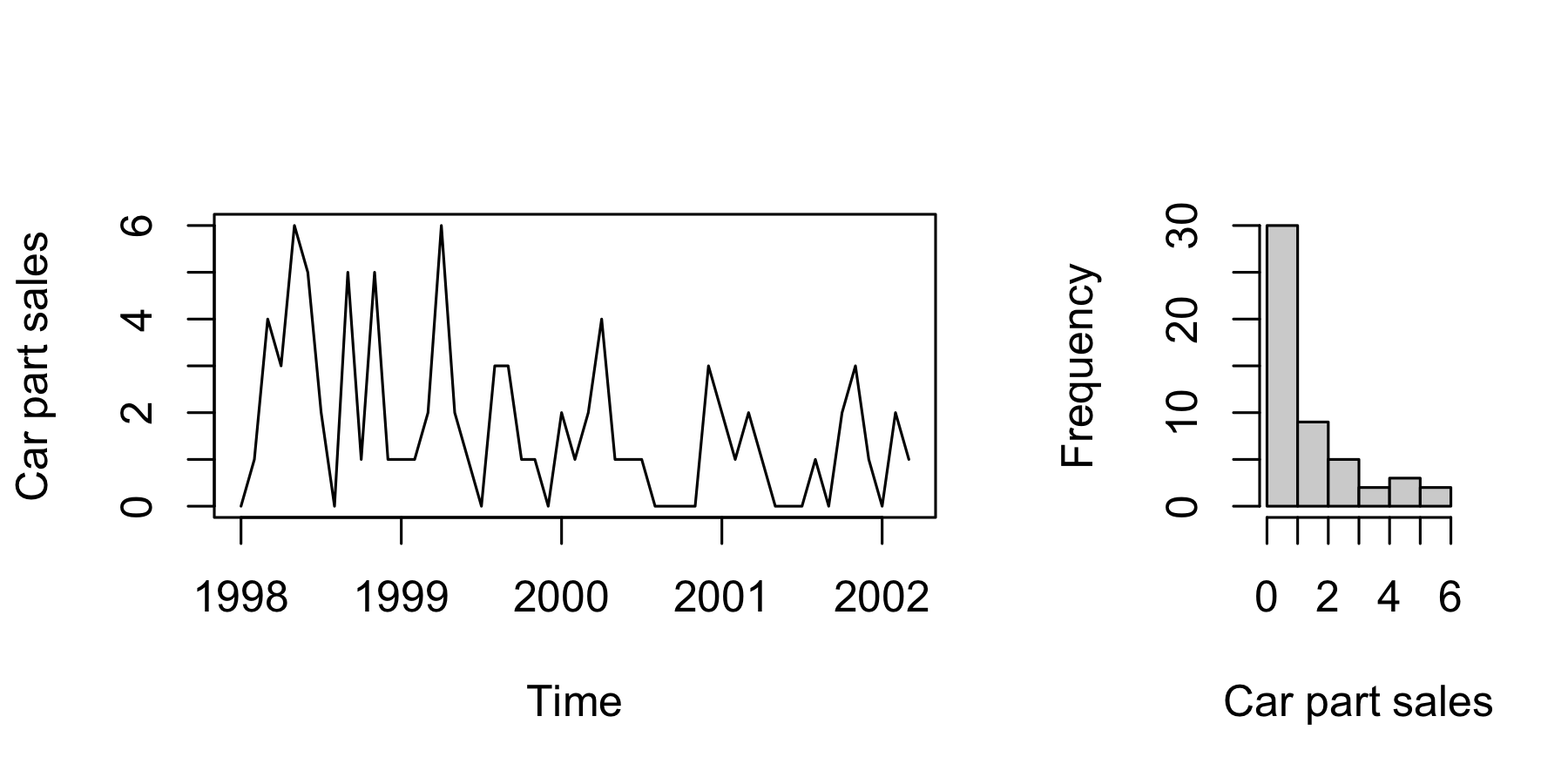Figure 1: Carpart - monthly car part sales.

We divide the time series into train and test; we want to forecast the test set, i.e., the last 12 observations (1 year ahead).

train <- window(carpart, end = c(2001, 3))
test <- window(carpart, start = c(2001, 4))

We now build the temporal hierarchy and compute the base forecasts.

We build the hierarchy using the temporal aggregation function. We aggregate at 2-Monthly, Quarterly, 4-Monthly, Biannual, and Annual by specifying the agg_levels argument.

train.agg <- bayesRecon::temporal_aggregation(train, agg_levels = c(2, 3, 4, 6, 12))
levels <- c("Annual", "Biannual", "4-Monthly", "Quarterly", "2-Monthly", "Monthly")
names(train.agg) <- levels

The function returns a list of aggregated time series, ordered from the top (most aggregated) to the bottom (most disagreggated).

par(mfrow = c(2, 3), mai = c(0.6, 0.6, 0.5, 0.5))
for (l in levels) {
plot(train.agg[[l]], xlab = "Time", ylab = "Car part sales", main = l)
}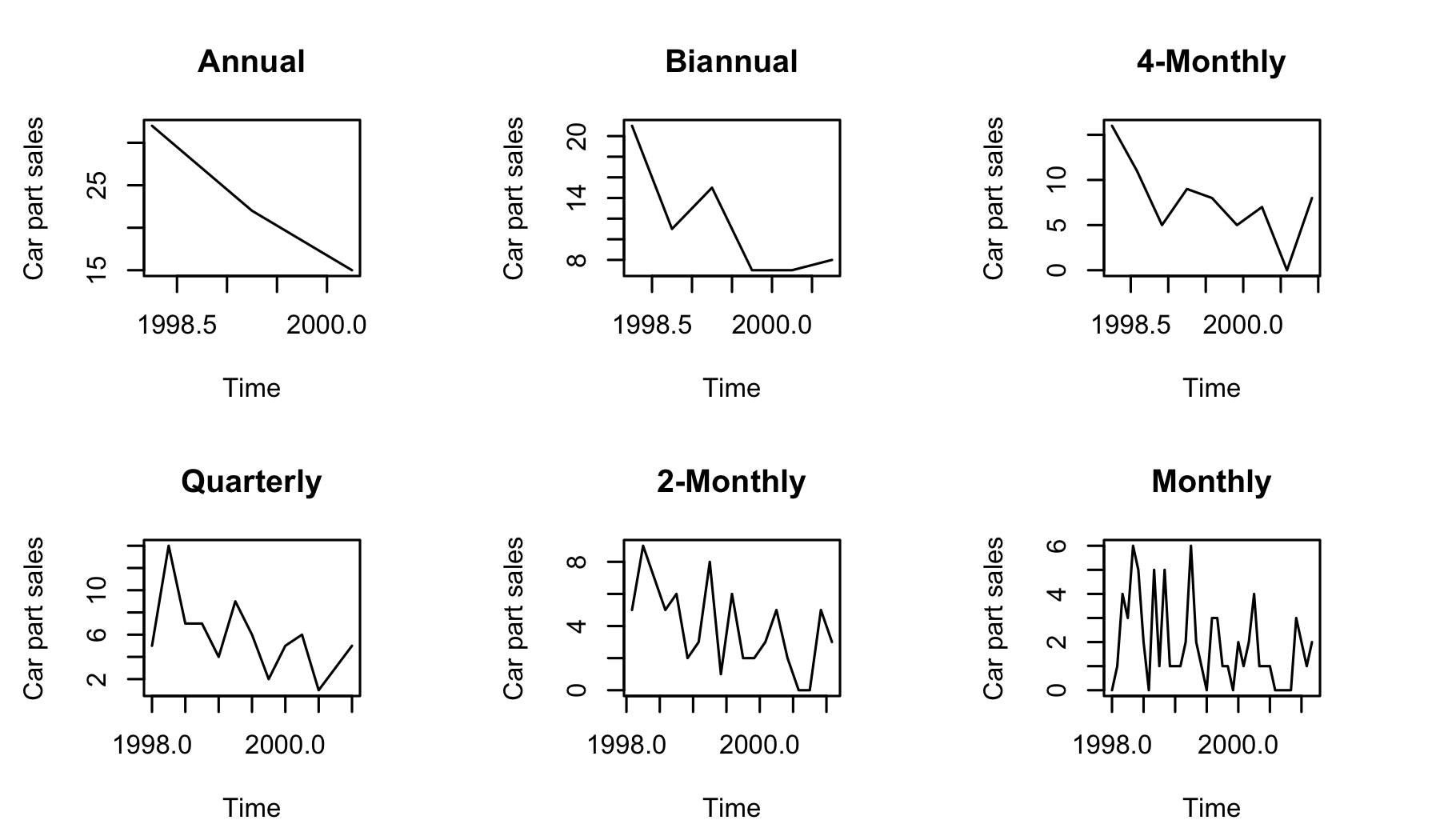Figure 2: Carpart - visualization of the aggregated time series.

We use the glarma package (R CRAN) to generate the forecasts. This package is specialized in forecasting count time series and it is based on a Generalized Linear Autoregressive Moving Average model. In this example, we adopt a Poisson predictive distribution.

The models produce forecasts for 1 year ahead; this is h=12 steps ahead for the monthly level, h=4 steps ahead for the quarterly level, etc. Each forecast is constituted by $$D$$ integer samples; we set $$D=20000$$.

Below we use a for loop to iterate over the levels of the temporal hierarchy. For each level, we fit an independent model; then we obtain the samples of the forecasts using the function glarma::forecast.glarma. Overall we have 28 predictive distributions (one for Annual, two for Biannual, etc.). We collect the samples of the 28 distributions in a list.

The following lines of code take ~ 30 seconds on a personal computer.

# install.packages("glarma", dependencies = TRUE)
#library(glarma)

fc.samples <- list()
D <- 20000
fc.count <- 1
# iterating over the temporal aggregation levels
for (l in seq_along(train.agg)) {
f.level <- frequency(train.agg[[l]])
print(paste("Forecasting at ", levels[l], "...", sep = ""))
# fit an independent model for each aggregation level
model <- glarma::glarma(
train.agg[[l]],
phiLags = if (f.level == 1) 1 else 1:(min(6, f.level - 1)),
thetaLags = if (f.level == 1) NULL else f.level,
X = cbind(intercept = rep(1, length(train.agg[[l]]))),
offset = cbind(intercept = rep(0, length(train.agg[[l]]))),
type = "Poi"
)
h <- f.level
tmp <- matrix(data = NA, nrow = h, ncol = D)
for (s in (1:D)) {
# each call to 'forecast.glarma' returns a simulation path
tmp[, s] <- glarma::forecast(
model,
newdata = cbind(intercept = rep(1, h)),
newoffset = rep(0, h)
)$Y } # collect the forecasted samples for (i in 1:h) { fc.samples[[fc.count]] <- tmp[i, ] fc.count <- fc.count + 1 } } #>  "Forecasting at Annual..." #>  "Forecasting at Biannual..." #>  "Forecasting at 4-Monthly..." #>  "Forecasting at Quarterly..." #>  "Forecasting at 2-Monthly..." #>  "Forecasting at Monthly..." Base forecasts do not sum up correctly. In order to address incoherence we can now use a reconciliation algorithm. First, we obtain the summing matrix $$\mathbf{S}$$ using the function get_reconc_matrices. It requires: • the aggregation factors used before to build the hierarchy, $$\{2, 3, 4, 6, 12\}$$ in this example; • the length of the forecasting horizon for the bottom time series (h=12 in this example). recon.matrices <- bayesRecon::get_reconc_matrices(agg_levels = c(2, 3, 4, 6, 12), h = 12) # Summing matrix S <- recon.matrices$S
# A matrix
A <- recon.matrices$A  Then, we use the function reconc_BUIS which implements the Bottom-Up Important Sampling (BUIS) algorithm. It requires the $$\mathbf{S}$$ matrix, the base forecasts, the type of the base forecasts (“samples” in this example) and the specification of the base forecasts distributions (“discrete” in this example, since the predictive distributions are Poisson). The in_type argument is a string with two possible values: “samples” if the base forecasts are in the form of samples, “params” if the base forecasts are in the form of estimated parameters. The distr argument is a string describing the type of base forecasts. It can be: “continuous” or “discrete” if in_type = "samples"; “gaussian”, “poisson” or “nbinom” if in_type = "params". recon.res <- bayesRecon::reconc_BUIS( S, base_forecasts = fc.samples, in_type = "samples", distr = "discrete", seed = 42 ) The function returns samples from the reconciled forecast distribution. reconciled_samples <- recon.res$reconciled_samples
dim(reconciled_samples)
#>     28 20000

We now compute the Mean Absolute Error (MAE) and the Continuous Ranked Probability Score (CRPS) for the bottom (i.e., monthly) time series. For CRPS, we use crps_sample from the package scoringRules (R CRAN).

# install.packages("scoringRules", dependencies = TRUE)
library(scoringRules)

ae.fc <- list()
ae.reconc <- list()
crps.fc <- list()
crps.reconc <- list()
for (h in 1:length(test)) {
y.hat_ <- median(fc.samples[[nrow(A) + h]])
y.reconc_ <- median(recon.res$bottom_reconciled_samples[, h]) # Compute Absolute Errors ae.fc[[h]] <- abs(test[h] - y.hat_) ae.reconc[[h]] <- abs(test[h] - y.reconc_) # Compute Continuous Ranked Probability Score (CRPS) crps.fc[[h]] <- scoringRules::crps_sample(y = test[h], dat = fc.samples[[nrow(A) + h]]) crps.reconc[[h]] <- scoringRules::crps_sample(y = test[h], dat = recon.res$bottom_reconciled_samples[, h])
}

mae.fc <- mean(unlist(ae.fc))
mae.reconc <- mean(unlist(ae.reconc))
crps.fc <- mean(unlist(crps.fc))
crps.reconc <- mean(unlist(crps.reconc))
metrics <- data.frame(
row.names = c("MAE", "CRPS"),
base.forecasts = c(mae.fc, crps.fc),
reconciled.forecasts = c(mae.reconc, crps.reconc)
)
metrics
#>      base.forecasts reconciled.forecasts
#> MAE       1.2500000            1.0416667
#> CRPS      0.7093284            0.6840278

Note the improvements with respect to the base forecast on both MAE and CRPS metrics.

# Example 2: M3

We now consider a monthly time series (N1485) from the M3 forecasting competition (Makridakis and Hibon 2000). It is available from bayesRecon::M3sample.

plot(M3example$train, xlab = "Time", ylab = "y", main = "N1485")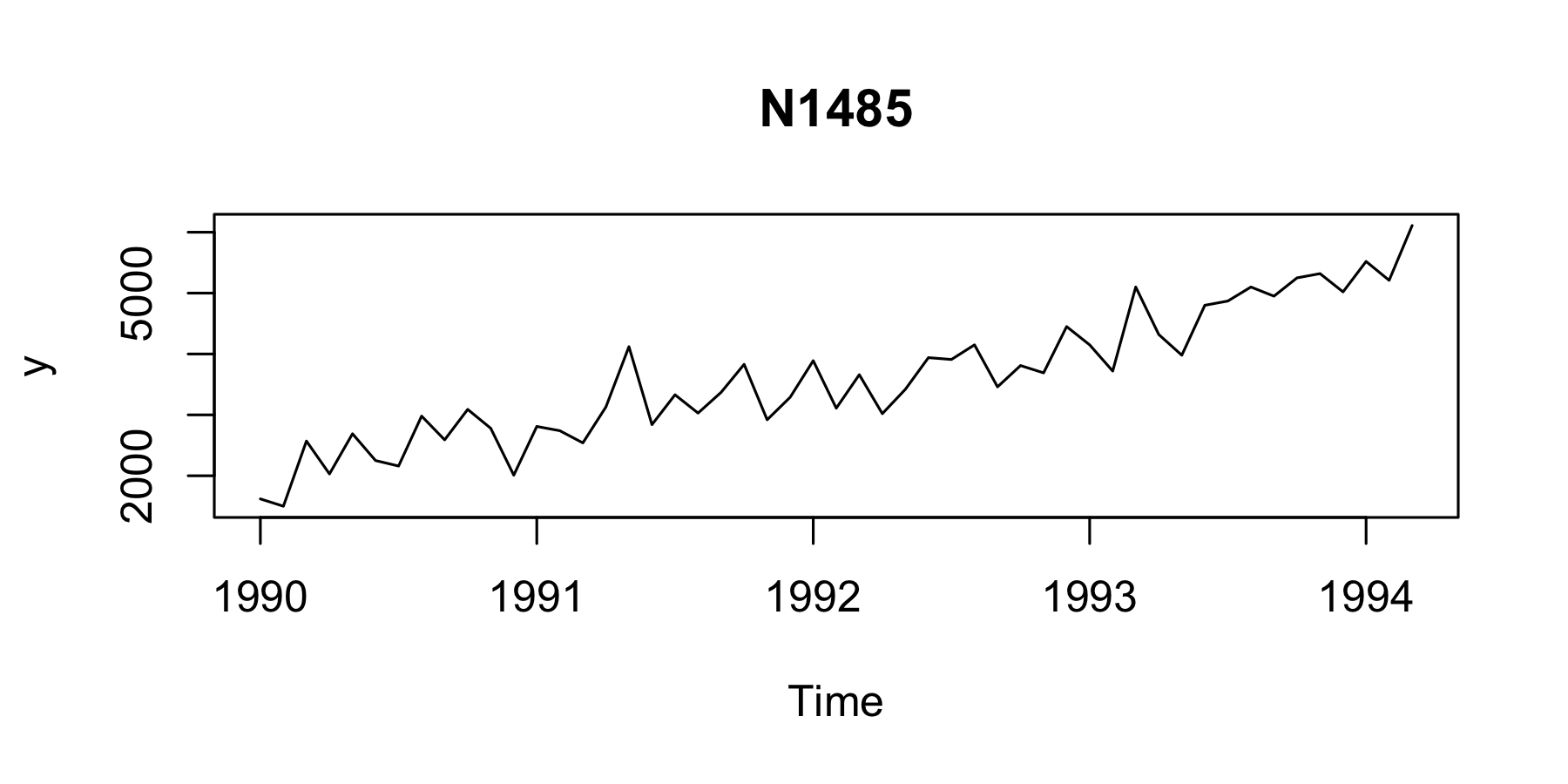Figure 3: M3 - N1485 time series. We build the temporal hierarchy using the temporal_aggregation function. We aggregate at 2-Monthly, Quarterly, 4-Monthly, Biannual, and Annual. As we do not specify the agg_levels argument, the function generates all the factors of the time series frequency (i.e. frequency(M3example$train) ).

train.agg <- bayesRecon::temporal_aggregation(M3example$train) levels <- c("Annual", "Biannual", "4-Monthly", "Quarterly", "2-Monthly", "Monthly") names(train.agg) <- levels We use the ets models from forecast (R CRAN) to generate forecast for each temporal level, for each h. The predictive distribution is Gaussian and we predict the next 18 months (the value of h is different for each temporal level). # install.packages("forecast", dependencies = TRUE) library(forecast) #> Registered S3 method overwritten by 'quantmod': #> method from #> as.zoo.data.frame zoo H <- length(M3example$test)
H
#>  18

fc <- list()
level.idx <- 1
fc.idx <- 1
for (level in train.agg) {
level.name <- names(train.agg)[level.idx]
# fit an ETS model for each temporal level
model <- ets(level)
# generate forecasts for each level within 18 months
h <- floor(H / (12 / frequency(level)))
print(paste("Forecasting at ", level.name, ", h=", h, "...", sep = ""))
level.fc <- forecast(model, h = h)
# save mean and sd of the gaussian predictive distribution
for (i in 1:h) {
fc[[fc.idx]] <- c(level.fc$mean[[i]], (level.fc$upper[, "95%"][[i]] - level.fc$mean[[i]]) / qnorm(0.975)) fc.idx <- fc.idx + 1 } level.idx <- level.idx + 1 } #>  "Forecasting at Annual, h=1..." #>  "Forecasting at Biannual, h=3..." #>  "Forecasting at 4-Monthly, h=4..." #>  "Forecasting at Quarterly, h=6..." #>  "Forecasting at 2-Monthly, h=9..." #>  "Forecasting at Monthly, h=18..." Before reconciling the forecasts, we obtain the matrix $$\mathbf{S}$$ using the function get_reconc_matrices. rmat <- get_reconc_matrices(agg_levels = c(2, 3, 4, 6, 12), h = 18) par(mai = c(1,1,0.5,0.5)) image(1:ncol(rmat$A), 1:nrow(rmat$A), t(apply(t(rmat$A),1,rev)),
xaxt='n', yaxt='n', ylab = "", xlab = levels)
axis(1, at=1:ncol(rmat$A), label=1:ncol(rmat$A), las=1)
axis(2, at=c(23,22,19,15,9), label=levels[1:5], las=2)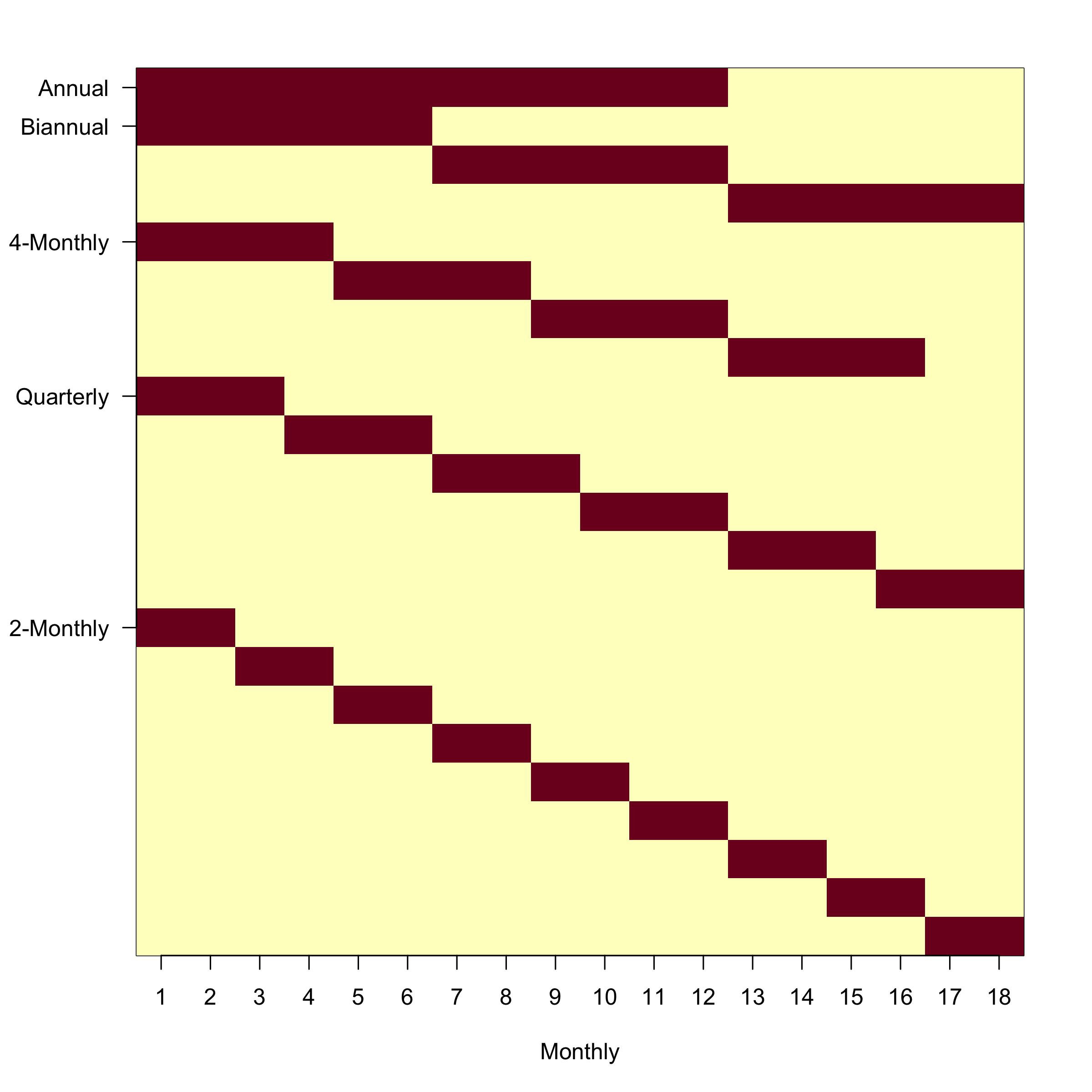Figure 4: M3 - The aggregating matrix A (red=1, yellow=0).

We now use the function reconc_gaussian which implements the closed-form Gaussian reconciliation, and the function reconc_BUIS which implements the Bottom-Up Important Sampling (BUIS) algorithm. We can check that the algorithms return consistent results, apart from minor numerical differences due to the sampling randomness of BUIS, which get smaller as we increase the num_samples argument of reconc_BUIS.

recon.gauss <- bayesRecon::reconc_gaussian(
S = rmat$S, base_forecasts.mu = sapply(fc, "[[", 1), base_forecasts.Sigma = diag(sapply(fc, "[[", 2)) ^ 2 ) reconc.buis <- bayesRecon::reconc_BUIS( S = rmat$S,
base_forecasts = fc,
in_type = "params",
distr = "gaussian",
num_samples = 20000,
seed = 42
)

# check that the algorithms return consistent results
round(rbind(
c(recon.gauss$upper_reconciled_mean, recon.gauss$bottom_reconciled_mean),
rowMeans(reconc.buis$reconciled_samples) )) #> [,1] [,2] [,3] [,4] [,5] [,6] [,7] [,8] [,9] [,10] [,11] [,12] #> [1,] 74977 35913 39063 41491 23520 25136 26321 27174 17464 18450 19251 19812 #> [2,] 74946 35897 39049 41470 23532 25091 26323 27134 17462 18435 19231 19818 #> [,13] [,14] [,15] [,16] [,17] [,18] [,19] [,20] [,21] [,22] [,23] [,24] #> [1,] 20412 21079 11527 11993 12393 12743 13047 13274 13531 13643 14317 5694 #> [2,] 20425 21046 11547 11985 12365 12726 13041 13282 13482 13652 14336 5694 #> [,25] [,26] [,27] [,28] [,29] [,30] [,31] [,32] [,33] [,34] [,35] [,36] #> [1,] 5833 5936 6056 6138 6255 6324 6419 6508 6538 6619 6655 6759 #> [2,] 5853 5914 6070 6127 6237 6308 6418 6505 6536 6612 6670 6729 #> [,37] [,38] [,39] [,40] [,41] #> [1,] 6772 6881 6762 7160 7157 #> [2,] 6753 6943 6709 7152 7184 We now show the base forecasts and the reconciled forecasts. yhat.mu <- tail(sapply(fc, "[[", 1), 18) yhat.sigma <- tail(sapply(fc, "[[", 2), 18) yhat.hi95 <- qnorm(0.975, mean = yhat.mu, sd = yhat.sigma) yhat.lo95 <- qnorm(0.025, mean = yhat.mu, sd = yhat.sigma) yreconc.mu <- rowMeans(reconc.buis$bottom_reconciled_samples)
yreconc.hi95 <- apply(reconc.buis$bottom_reconciled_samples, 1, function(x) quantile(x, 0.975)) yreconc.lo95 <- apply(reconc.buis$bottom_reconciled_samples, 1,
function(x) quantile(x, 0.025))

ylim_ <- c(min(M3example$train, M3example$test, yhat.lo95, yreconc.lo95) - 1,
max(M3example$train, M3example$test, yhat.hi95, yreconc.hi95) + 1)

plot(M3example$train, xlim = c(1990, 1995.6), ylim = ylim_, ylab = "y", main = "N1485 Forecasts") lines(M3example$test, lty = "dashed")
lines(ts(yhat.mu, start = start(M3example$test), frequency = 12), col = "coral", lwd = 2) lines(ts(yreconc.mu, start = start(M3example$test), frequency = 12),
col = "blue2", lwd = 2)
xtest <- time(M3example$test) polygon(c(xtest, rev(xtest)), c(yhat.mu, rev(yhat.hi95)), col = "#FF7F5066", border = "#FF7F5066") polygon(c(xtest, rev(xtest)), c(yhat.mu, rev(yhat.lo95)), col = "#FF7F5066", border = "#FF7F5066") polygon(c(xtest, rev(xtest)), c(yreconc.mu, rev(yreconc.hi95)), col = "#0000EE4D", border = "#0000EE4D") polygon(c(xtest, rev(xtest)), c(yreconc.mu, rev(yreconc.lo95)), col = "#0000EE4D", border = "#0000EE4D")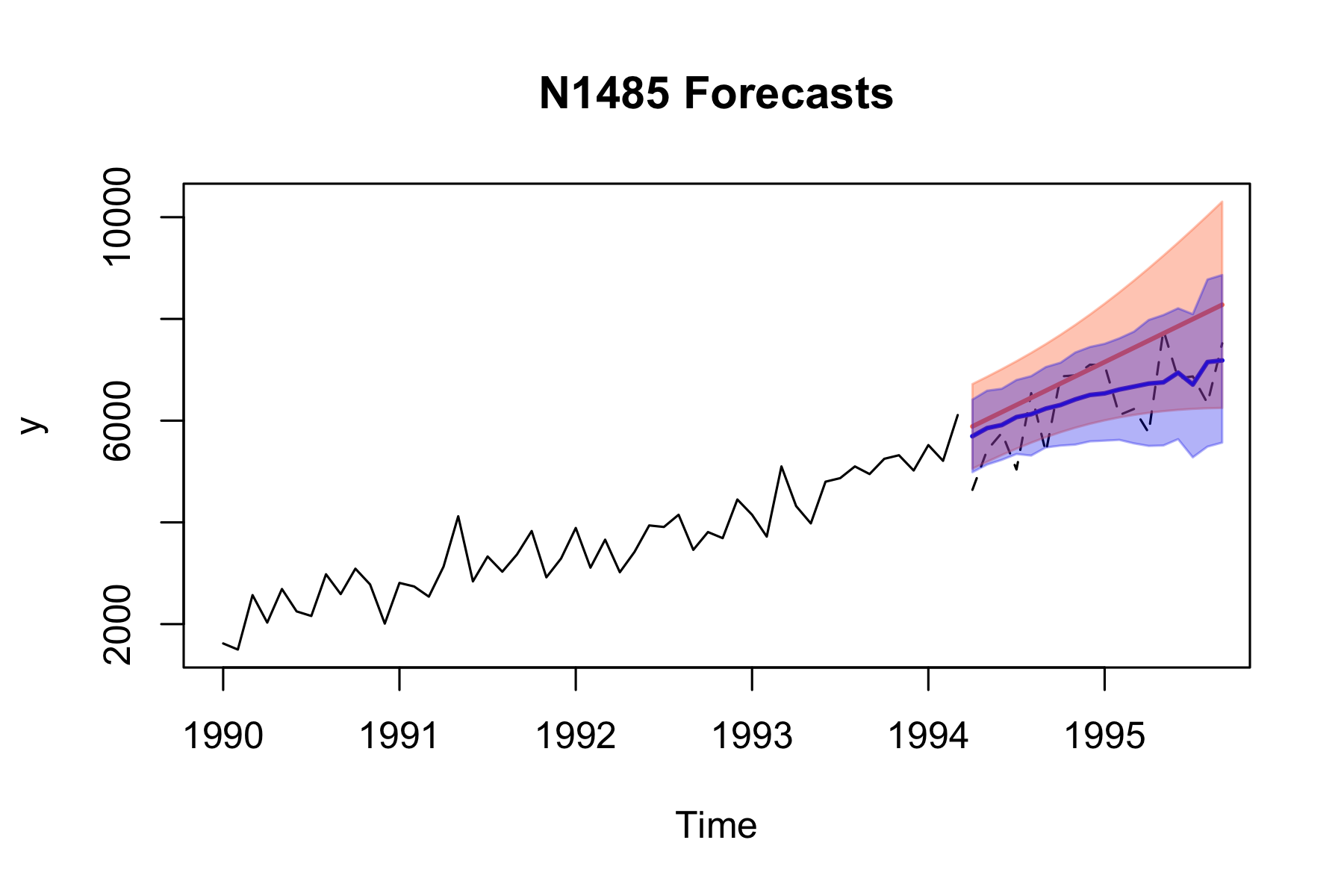Figure 5: M3 - visualization of base and reconciled forecasts. The black line is the actual data (dashed is the test). The orange line is the forecasted mean, the blu line the reconciled mean. Shadow regions show the 95% prediction intervals. # Example 3: Infants mortality In this example, we consider the infantgts hierarchical time series (R. J. Hyndman et al. 2011). It is available via bayesRecon::infantMortality. It is a yearly grouped cross-sectional time series dataset, from 1901 to 2003, of infant mortality counts (deaths) in Australia, disaggregated by state and sex (male and female). State codes refer to: New South Wales (NSW), Victoria (VIC), Queensland (QLD), South Australia (SA), Western Australia (WA), Northern Territory (NT), Australian Capital Territory (ACT), and Tasmania (TAS). We want to forecast the next year, 2004. We use the auto.arima forecasting models from the package forecast (R CRAN) to generate Gaussian predictions for each node of the hierarchy. For each model, we collect the residuals to take into account correlations. # install.packages("forecast", dependencies = TRUE) library(forecast) fc <- list() residuals <- matrix(NA, nrow = length(infantMortality$total),
ncol = length(infantMortality))
fc.idx <- 1
for (s in infantMortality) {
s.name <- names(infantMortality)[fc.idx]
print(paste("Forecasting at ", s.name, "...", sep = ""))
# fit an auto.arima model and forecast with h=1
model <- auto.arima(s)
s.fc <- forecast(model, h = 1)
# save mean and sd of the gaussian predictive distribution
fc[[s.name]] <- c(s.fc$mean, (s.fc$upper[, "95%"][] - s.fc$mean) / qnorm(0.975)) residuals[, fc.idx] <- s.fc$residuals
fc.idx <- fc.idx + 1
}
#>  "Forecasting at total..."
#>  "Forecasting at NSW..."
#>  "Forecasting at VIC..."
#>  "Forecasting at QLD..."
#>  "Forecasting at SA..."
#>  "Forecasting at WA..."
#>  "Forecasting at NT..."
#>  "Forecasting at ACT..."
#>  "Forecasting at TAS..."
#>  "Forecasting at male..."
#>  "Forecasting at female..."
#>  "Forecasting at NSW male..."
#>  "Forecasting at NSW female..."
#>  "Forecasting at VIC male..."
#>  "Forecasting at VIC female..."
#>  "Forecasting at QLD male..."
#>  "Forecasting at QLD female..."
#>  "Forecasting at SA male..."
#>  "Forecasting at SA female..."
#>  "Forecasting at WA male..."
#>  "Forecasting at WA female..."
#>  "Forecasting at NT male..."
#>  "Forecasting at NT female..."
#>  "Forecasting at ACT male..."
#>  "Forecasting at ACT female..."
#>  "Forecasting at TAS male..."
#>  "Forecasting at TAS female..."

Build the $$\mathbf{S}$$ matrix.

# we have 16 bottom time series, and 11 upper time series
A <- matrix(data = c(1,1,1,1,1,1,1,1,1,1,1,1,1,1,1,1,
1,1,0,0,0,0,0,0,0,0,0,0,0,0,0,0,
0,0,1,1,0,0,0,0,0,0,0,0,0,0,0,0,
0,0,0,0,1,1,0,0,0,0,0,0,0,0,0,0,
0,0,0,0,0,0,1,1,0,0,0,0,0,0,0,0,
0,0,0,0,0,0,0,0,1,1,0,0,0,0,0,0,
0,0,0,0,0,0,0,0,0,0,1,1,0,0,0,0,
0,0,0,0,0,0,0,0,0,0,0,0,1,1,0,0,
0,0,0,0,0,0,0,0,0,0,0,0,0,0,1,1,
1,0,1,0,1,0,1,0,1,0,1,0,1,0,1,0,
0,1,0,1,0,1,0,1,0,1,0,1,0,1,0,1), byrow=TRUE, ncol = 16)
S <- rbind(A, diag(16))

# plot of A
par(mai = c(1.5,1,0.5,0.5))
image(1:ncol(A), 1:nrow(A),
t(apply(t(A),1,rev)),
xaxt='n', yaxt='n', ann=FALSE)
axis(1, at=1:ncol(A), label=names(infantMortality)[12:27], las=2)
axis(2, at=c(1:11), label=rev(names(infantMortality)[1:11]), las=2)